# 2 k3 2 3 4 a. (10 points) Obtain the global stiffness matrix K using direct...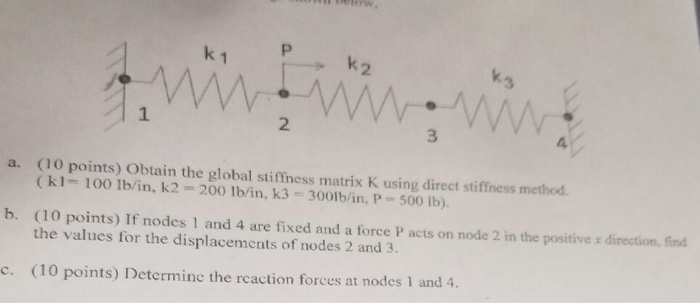2 k3 2 3 4 a. (10 points) Obtain the global stiffness matrix K using direct stiffness method (k1- 100 1b/in, k2 200 lb/in, k3 3001b/in, P-500 Ib). (10 points) If nodes 1 and 4 are fixed and a force P acts on node 2 in the positive x direction, fin the values for the displacements of nodes 2 and 3. b. c. (10 points) Deter odes 1 and 4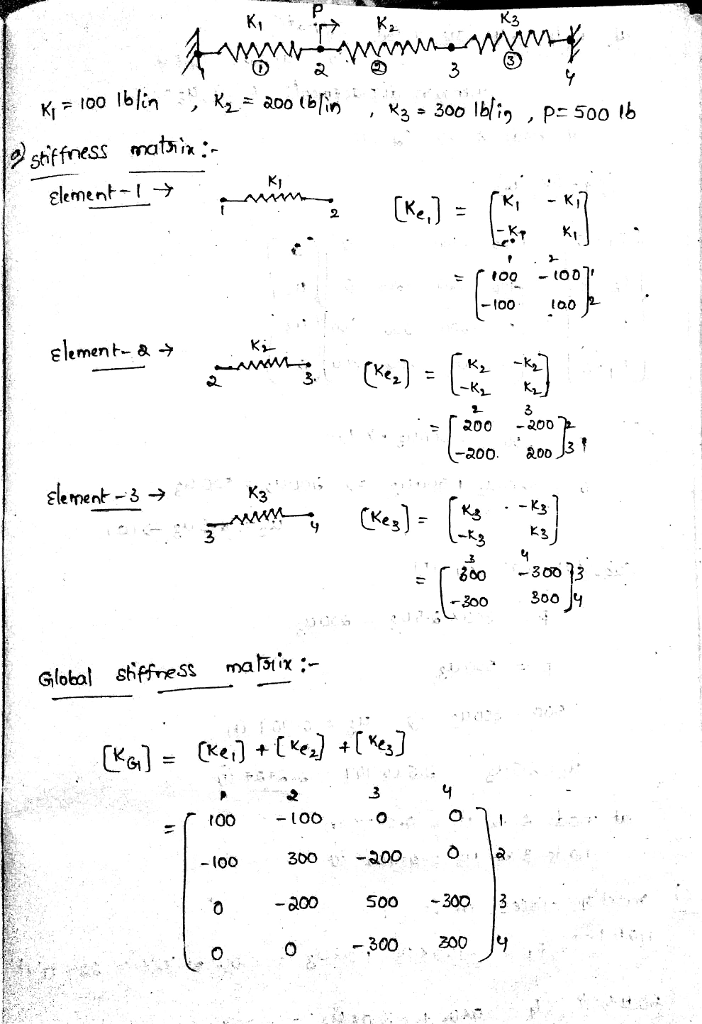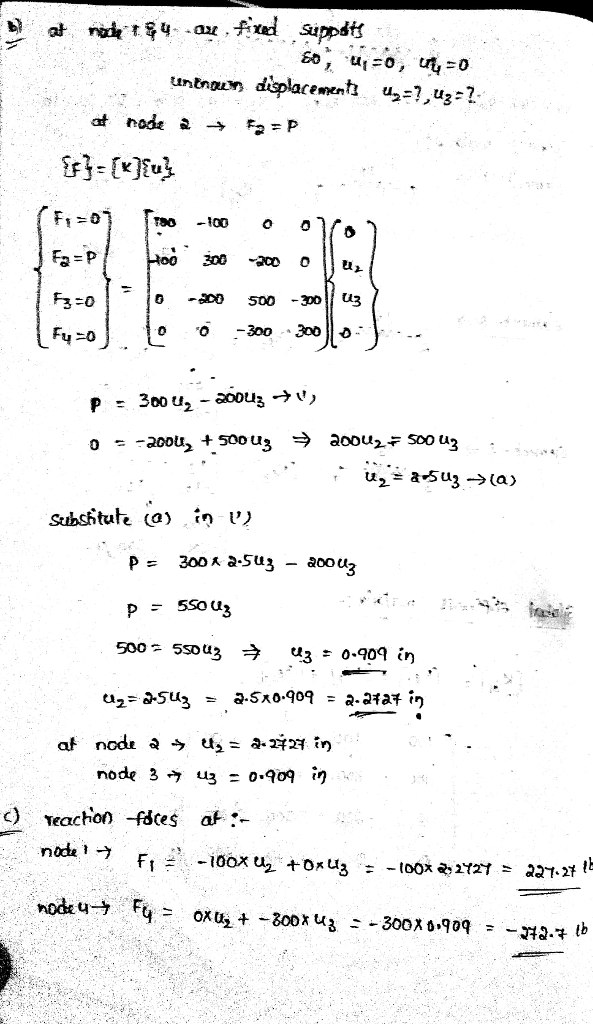#### Earn Coin

Coins can be redeemed for fabulous gifts.

Similar Homework Help Questions
• ### 5. For the one-dimensional problem shown below, calculate: a. The global stiffness matrix before the application...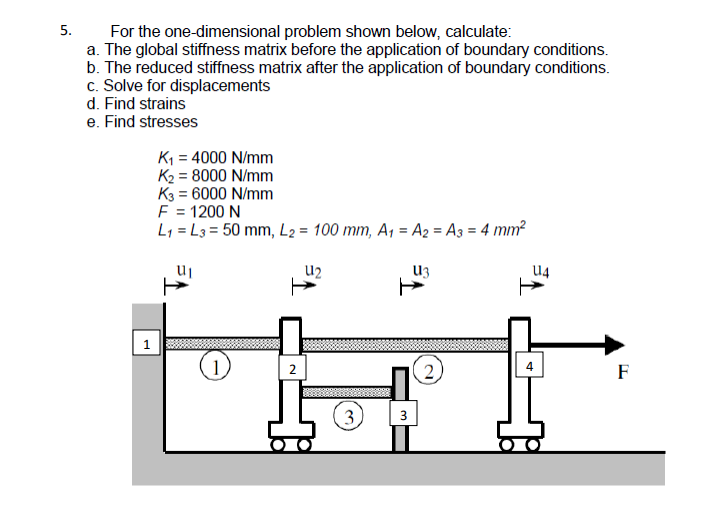5. For the one-dimensional problem shown below, calculate: a. The global stiffness matrix before the application of boundary conditions. b. The reduced stiffness matrix after the application of boundary conditions. c. Solve for displacements d. Find strains e. Find stresses K1 = 4000 N/mm K2 = 8000 N/mm K3 = 6000 N/mm F = 1200 N L1 = L3 = 50 mm, L2 = 100 mm, A1 = A2 = A3 = 4 mm2 SWASTA 888888888888

• ### Given condition: k1=k2=k3=k4=k5=k,F3=P, and nodes 1,2,5 are fixed Decision a.Global stiffness matrix b. Displacement of nodes...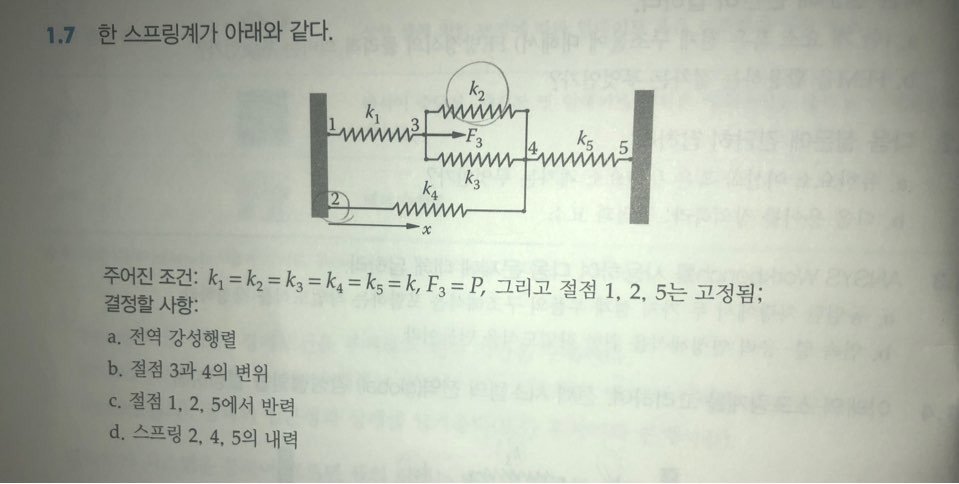Given condition: k1=k2=k3=k4=k5=k,F3=P, and nodes 1,2,5 are fixed Decision a.Global stiffness matrix b. Displacement of nodes 3 and 4 c. Reaction force at nodes 1,2,5 d.Internal force of spring 2,4,5 Oh, and I would appreciate it if you could tell the answer using computer typing instead of handwriting. Because I don't know the cursive If it is difficult, I would appreciate it if you could respond in a convenient way. 1.7 한 스프링계가 아래와 같다. k2 ki -F3 Amino k4...

• ### 1. Find displacements at each node and forces in each element for the series of spring...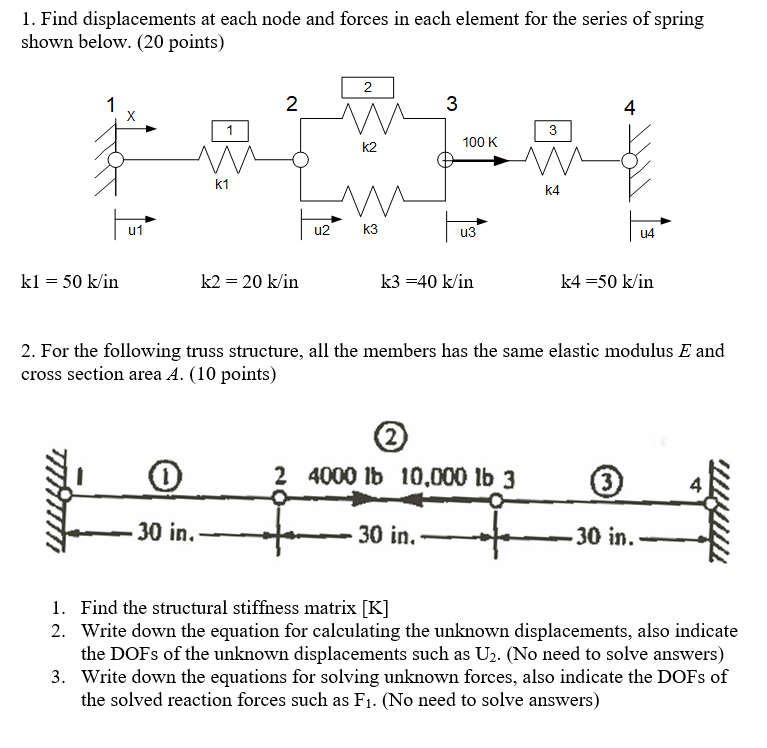1. Find displacements at each node and forces in each element for the series of spring shown below. (20 points) 3 4 3 100 K k1 k4 u2 k3 из U4 k1 50 k/in k2- 20 k/in k3-40 k/in k4-50 k/in 2. For the following truss structure, all the members has the same elastic modulus E and cross section area A. (10 points) 2 4000 lb 10.000 lb 3 3 4 30 in. 30 in. 30 in Find the structural...

• ### For the system shown below, (a) the global stiffness matrix   (b)displacements of nodes 2 and 3...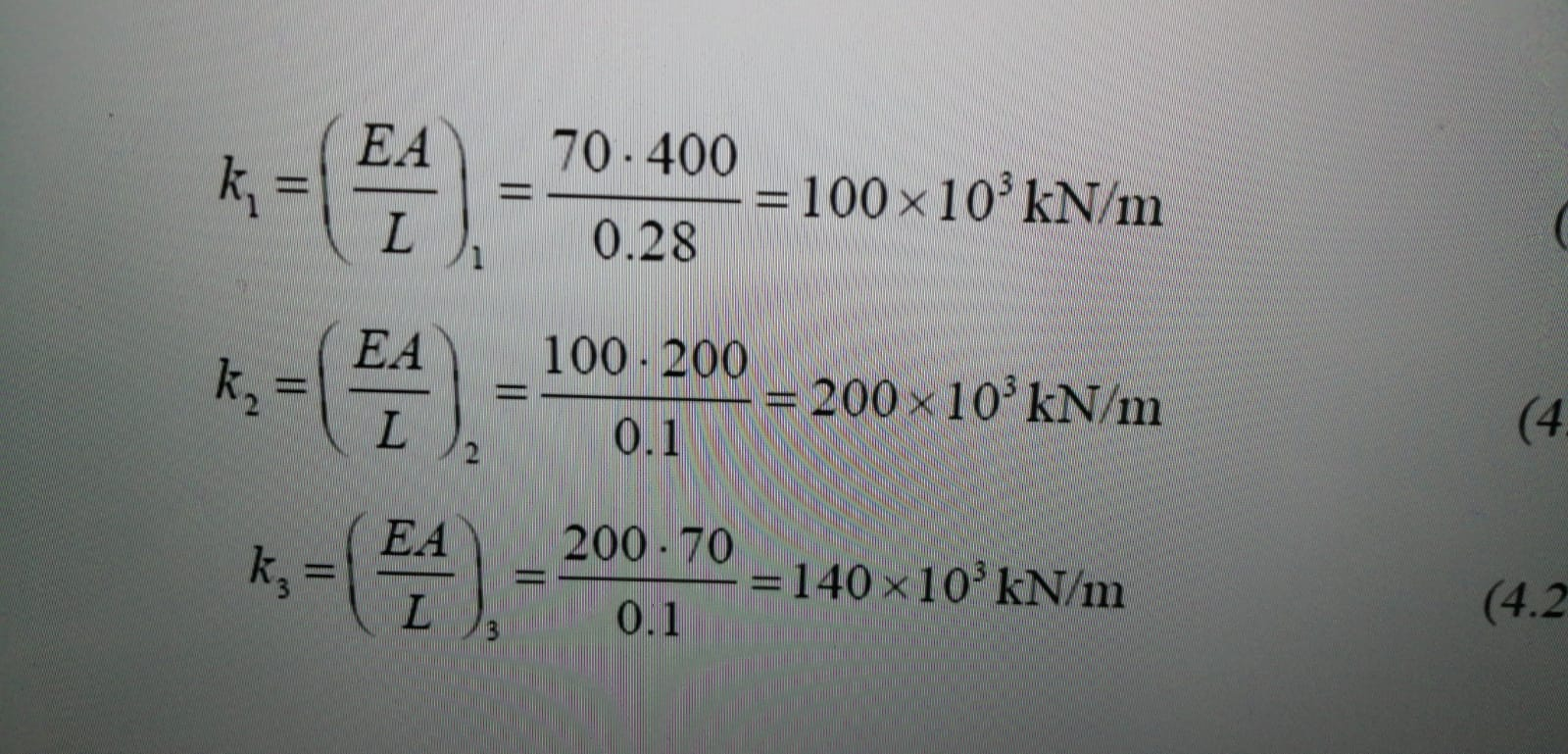For the system shown below, (a) the global stiffness matrix   (b)displacements of nodes 2 and 3 (c)the reaction forces at nodes 1 and 4 (d)the force in the members EA TRATAMI 70-400 = 100 x 10 kN/m 0.28 ATT L ( EA k, 100 - 200 = 200 x 10 kN/m 0.1 L (4 EA k, 200.70 =140x10 kN/m 0.1 I (4.2 X tretiet 0.28 2 vyos Imool

• ### Three rigid bodies (Nodes 2, 3, and 4) are connected by five springs as shown below....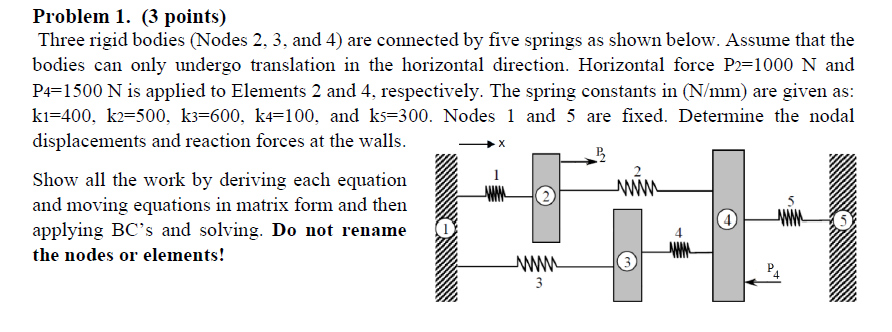Three rigid bodies (Nodes 2, 3, and 4) are connected by five springs as shown below. Assume that the bodies can only undergo translation in the horizontal direction. Horizontal force P2=1000 N and P4=1500 N is applied to Elements 2 and 4, respectively. The spring constants in (N/mm) are given as: k1=400, k2=500, k3=600, k4=100, and k5=300. Nodes 1 and 5 are fixed. Determine the nodal displacements and reaction forces at the walls. Problem 1. (3 points) Three rigid bodies...

• ### a. Compute the total stiffness matrix [K] of the assemblage shown in Figure 3-1 by superimposing...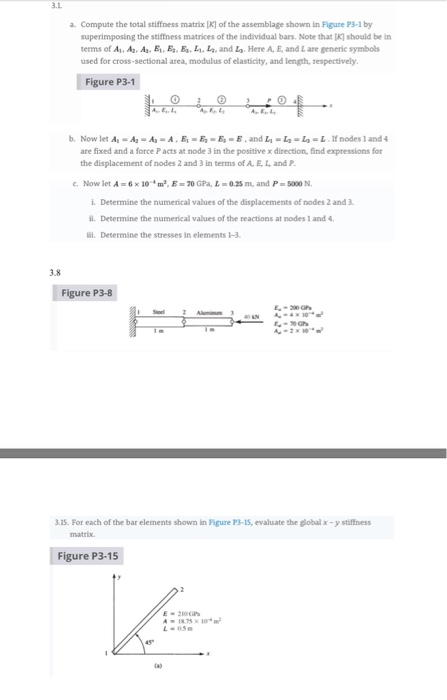a. Compute the total stiffness matrix [K] of the assemblage shown in Figure 3-1 by superimposing the stiffness matrices of the individual bars. Note that should be in terms of A. As, A, E, E E, L. and L. Here A, E, and are generic symbols used for cross-sectional area modulus of elasticity, and length, respectively Figure P3-1 Now let As - Ag-A-A.E E, E E and L-L L -L nodes 1 and 4 are fixed and a force Pacts...

• ### Problem 3 (23%) The following beam is discretize into 2 elements. E-29x106 psi, I-375 in and A (b...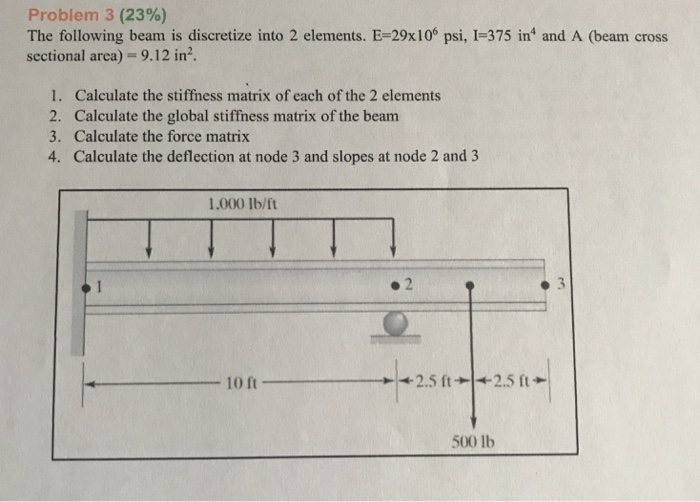Problem 3 (23%) The following beam is discretize into 2 elements. E-29x106 psi, I-375 in and A (beam cross sectional area) -9.12 in2 1. 2. 3. 4. Calculate the stiffness matrix of each of the 2 elements Calculate the global stiffness matrix of the beam Calculate the force matrix Calculate the deflection at node 3 and slopes at node 2 and 3 1.000 lb/ft 10 ft .5 ft+-2.5 ft 500 lb Problem 3 (23%) The following beam is discretize into...

• ### i need help with c and d but explain why Question 1 (10 marks). Assembly A model consists of two 1D trusses with dimens...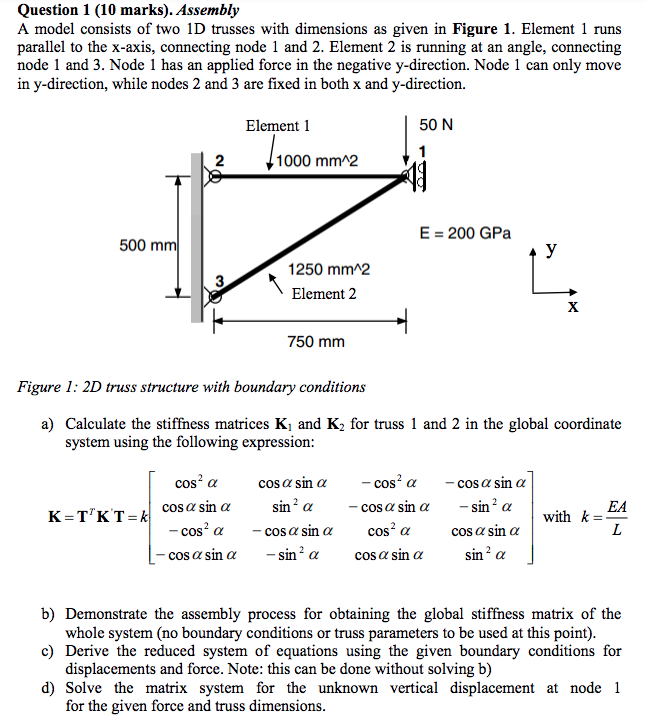i need help with c and d but explain why Question 1 (10 marks). Assembly A model consists of two 1D trusses with dimensions as given in Figure 1. Element 1 runs angle, connecting parallel to the x-axis, connecting node 1 and 2. Element 2 is running at an node 1 and 3. Node 1 has an applied force in the negative y-direction. Node 1 can only in y-direction, while nodes 2 and 3 are fixed in both x and...

• ### 2. For the pin-jointed truss shown in Figure Q2.1 applied at node 4. The Young's modulus E(GPa) is the same for...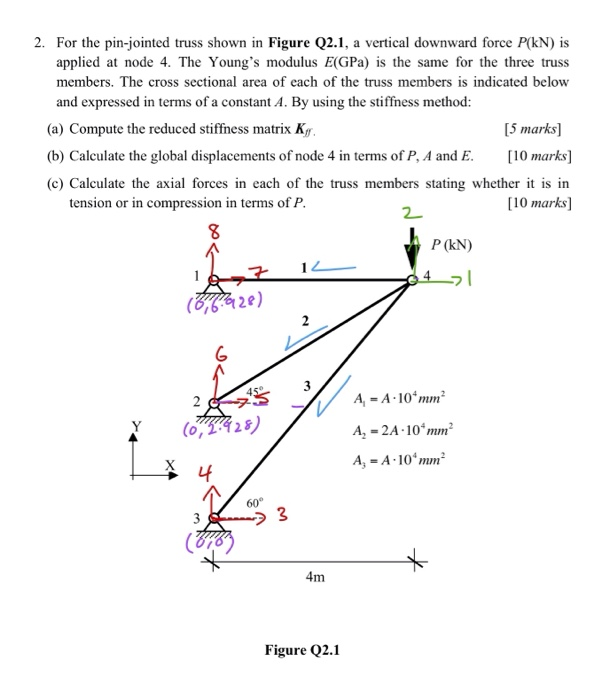2. For the pin-jointed truss shown in Figure Q2.1 applied at node 4. The Young's modulus E(GPa) is the same for the three truss vertical downward force P(kN) is a members. The cross sectional area of each of the truss members is indicated below and expressed in terms of a constant A. By using the stiffness method: (a) Compute the reduced stiffness matrix Kg [5 marks [10 marks (b) Calculate the global displacements of node 4 in terms of P,...

• ### Week 9. Question 1: Use the stiffness method to analyse the structure shown below. For the...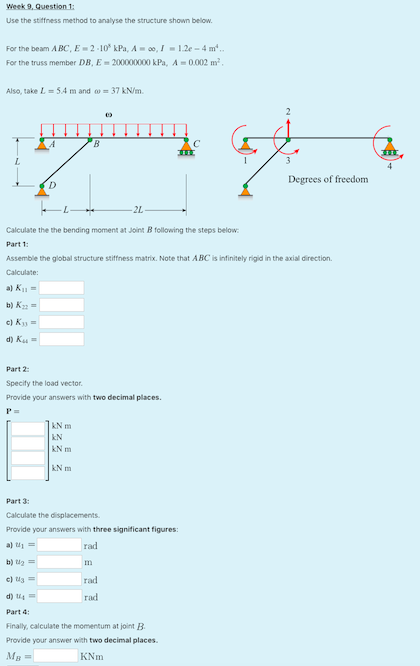Week 9. Question 1: Use the stiffness method to analyse the structure shown below. For the beam ABC, E = 2 -10% kPa, A -00, = 1.2e - 4 m. For the truss member DB, E = 200000000 kPa, A = 0.002 m. Also, take L54 m and w37 kN/m с 7 Degrees of freedom 22 Calculate the the bending moment at Joint B following the steps below. Part 1: Assemble the global structure stiffness matrix. Note that ABC is...